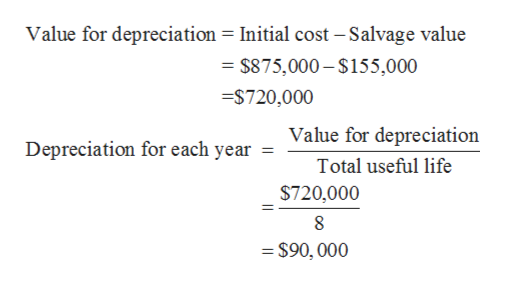# Your company is considering a new project that will require \$875,000 of new equipment at the start of the project. The equipment will have a depreciable life of 8 years and will be depreciated to a book value of \$155,000 using straight-line depreciation. The cost of capital is 11 percent, and the firm’s tax rate is 30 percent.

Question
8 views

Your company is considering a new project that will require \$875,000 of new equipment at the start of the project. The equipment will have a depreciable life of 8 years and will be depreciated to a book value of \$155,000 using straight-line depreciation. The cost of capital is 11 percent, and the firm’s tax rate is 30 percent.

check_circle

Step 1

It is assumed that the present value of tax benefits from the new projects is required to be calculated which help an investor to evaluate the project or investment.

Step 2

Given:

Initial cost of the new project = \$875,000

Useful life is 8 years

Salvage or residual value is \$155,000

The cost of capital is 11%

Tax rate is 30%

Step 3

Firstly, depreciation wi...help_outlineImage TranscriptioncloseValue for depreciation = Initial cost -Salvage value = \$875,000-\$155,000 =\$720,000 Value for depreciation Depreciation for each year Total useful life \$720,000 8 = \$90, 000 fullscreen

### Want to see the full answer?

See Solution

#### Want to see this answer and more?

Solutions are written by subject experts who are available 24/7. Questions are typically answered within 1 hour.*

See Solution
*Response times may vary by subject and question.
Tagged in